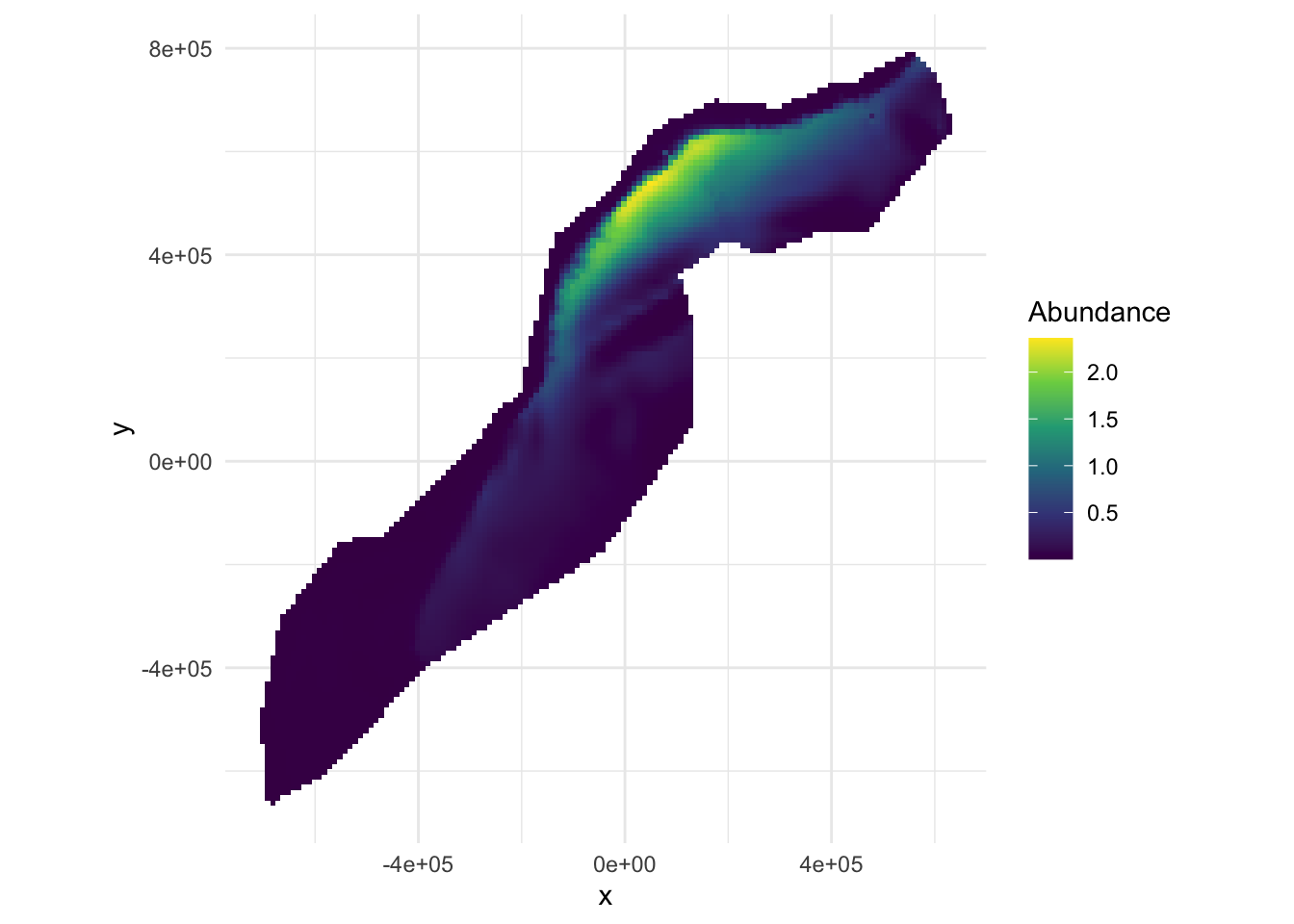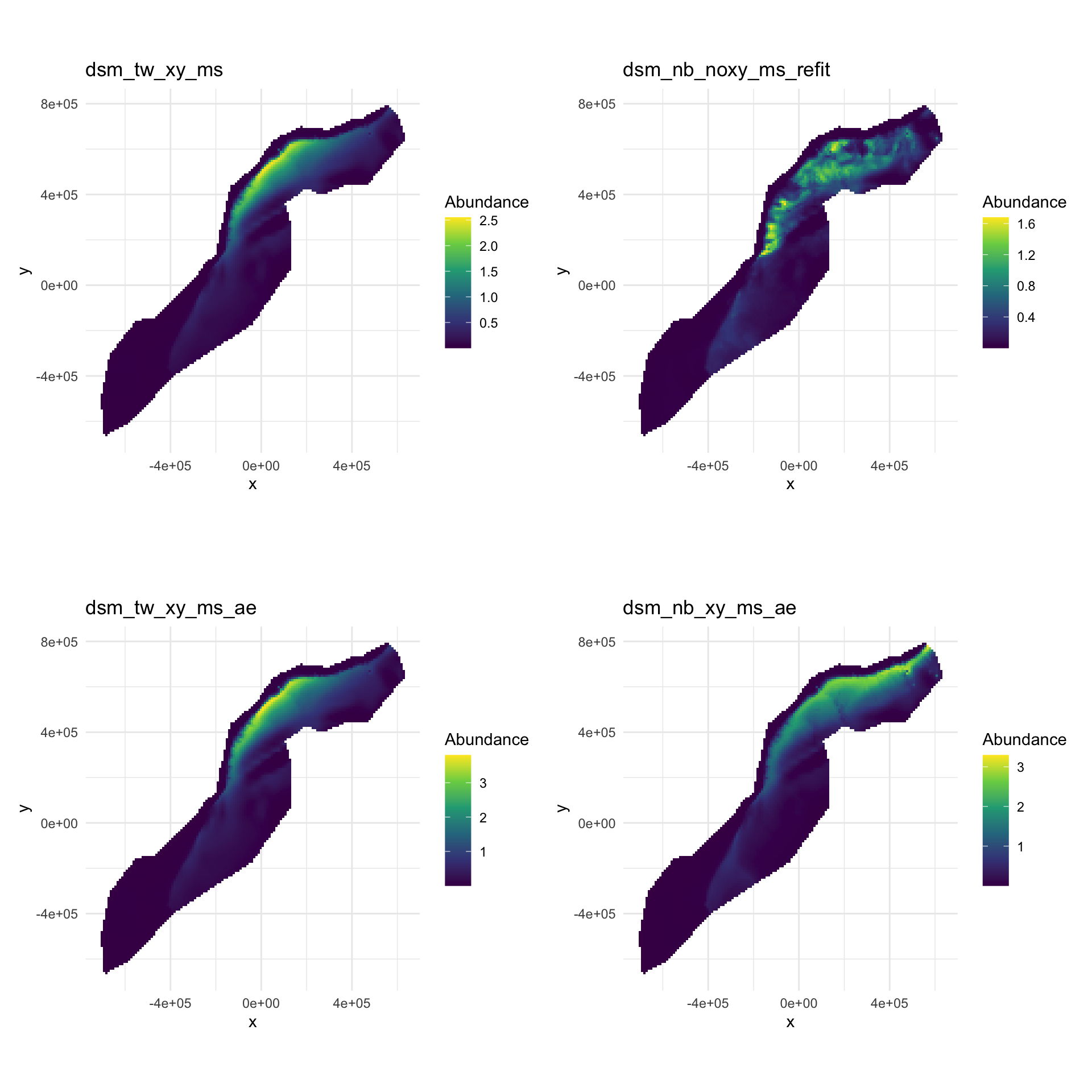## Aims

By the end of this practical, you should feel comfortable:

• Making a prediction using the `predict()` function
• Summing the prediction cells to obtain a total abundance for a given area
• Plotting a map of predictions
• Knowing when to use `dsm.var.prop` and when to use `dsm.var.gam`
• Estimating variance for a given prediction area
• Estimating variance per-cell for a prediction grid
• Interpreting the `summary()` output for uncertainty estimates
• Making maps of the coefficient of variation in R

``````library(knitr)
library(dsm)``````
``## Loading required package: mgcv``
``## Loading required package: nlme``
``## This is mgcv 1.8-33. For overview type 'help("mgcv-package")'.``
``## Loading required package: mrds``
``````## This is mrds 2.2.3
## Built: R 4.0.2; ; 2020-08-01 10:33:56 UTC; unix``````
``## Loading required package: numDeriv``
``````## This is dsm 2.3.0
## Built: R 4.0.2; ; 2020-07-16 23:56:50 UTC; unix``````
``````library(ggplot2)
library(patchwork)``````

``````load("spermwhale.RData")
# rename one model
dsm_nb_xy_ms <- dsm_nb_xy_ms_p1_4``````

## Prediction data

Our prediction data lives in the `predgrid` object. This data was prepared from various sources and the since some of the covariates were dynamic (SST, NPP and EKE), the dates for these were arbitrarily chosen as 2 June 2004, or Julian date 153.

If you’re preparing this data yourself, the `rerddap` package can be used to obtain marine data from NOAA for both segments and predictions.

We can take a look at our data using `head()` to see the first few rows:

``head(predgrid)``
``````##            x      y      Depth      SST      NPP DistToCAS          EKE off.set
## 126 547984.6 788254  153.59825 12.04609 1462.521 11788.974 0.0008329031   1e+08
## 127 557984.6 788254  552.31067 12.81379 1465.410  5697.248 0.0009806611   1e+08
## 258 527984.6 778254   96.81992 12.90251 1429.432 13722.626 0.0011575423   1e+08
## 259 537984.6 778254  138.23763 13.21393 1424.862  9720.671 0.0013417297   1e+08
## 260 547984.6 778254  505.14386 13.75655 1379.351  8018.690 0.0026881567   1e+08
## 261 557984.6 778254 1317.59521 14.42525 1348.544  3775.462 0.0045683752   1e+08
##          long      lat
## 126 -66.52252 40.94697
## 127 -66.40464 40.94121
## 258 -66.76551 40.86781
## 259 -66.64772 40.86227
## 260 -66.52996 40.85662
## 261 -66.41221 40.85087``````

As we saw in the slides, the `predict()` function will fill in the variables in the model formula with these values to obtain the predictions at each cell.

For example using the model `dsm_nb_xy_ms`:

``pp <- predict(dsm_nb_xy_ms, predgrid, off.set=predgrid\$off.set)``

This is just a list of numbers – the predicted abundance per cell. We can sum these to get the estimated abundance for the study area:

``sum(pp, na.rm=TRUE)``
``##  1580.121``

We can also plot this to get a spatial representation of the predictions:

``````# assign the predictions to the prediction grid data.frame
predgrid\$Nhat_nb_xy <- pp

# plot!
p <- ggplot(predgrid) +
geom_tile(aes(x=x, y=y, fill=Nhat_nb_xy, width=10*1000, height=10*1000)) +
coord_equal() +
# better colour scheme
scale_fill_viridis_c() +
# cleaner plot
theme_minimal() +
# set the label for the fill colour
labs(fill="Abundance")
print(p)``````Predicted surface for abundance estimates with bivariate spatial smooth along with environmental covariates.

Use the chunks above as a template and make predictions for the other models you saved in the previous exercises. In particular, compare the models with only spatial terms to those with environmental covariates included.

Making predictions

Let’s try making predictions with a few models. Let’s compare two count models (negative binomial and Tweedie) an two estimated abundance models (negative binomial and Tweedie).

I’ll make the plots then use the `patchwork` package to add together plots and put them together at the end.

First the Tweedie count, with a smooth of space.

``````predgrid\$Nhat_tw_xy <- predict(dsm_tw_xy_ms, predgrid,
off.set=predgrid\$off.set)

# plot
p_tw_xy <- ggplot(predgrid) +
geom_tile(aes(x=x, y=y, fill=Nhat_tw_xy,
width=10*1000, height=10*1000)) +
coord_equal() +
scale_fill_viridis_c() +
theme_minimal() +
ggtitle("dsm_tw_xy_ms") +
labs(fill="Abundance")``````

Now make a plot for the negative binomial habitat count model:

``````predgrid\$Nhat_nb_noxy <- predict(dsm_nb_noxy_ms_refit,
predgrid,
off.set=predgrid\$off.set)

# plot
p_nb_noxy <- ggplot(predgrid) +
geom_tile(aes(x=x, y=y, fill=Nhat_nb_noxy,
width=10*1000, height=10*1000)) +
coord_equal() +
scale_fill_viridis_c() +
theme_minimal() +
ggtitle("dsm_nb_noxy_ms_refit") +
labs(fill="Abundance")``````

Now the estimated abundance, with negative binomial response:

``````predgrid\$Nhat_nb_ae <- predict(dsm_nb_xy_ms_ae, predgrid,
off.set=predgrid\$off.set)

# plot
p_nb_ae <- ggplot(predgrid) +
geom_tile(aes(x=x, y=y, fill=Nhat_nb_ae,
width=10*1000, height=10*1000)) +
coord_equal() +
scale_fill_viridis_c() +
theme_minimal() +
ggtitle("dsm_nb_xy_ms_ae") +
labs(fill="Abundance")``````

Finally estimated abundance, with Tweedie response:

``````predgrid\$Nhat_tw_ae <- predict(dsm_tw_xy_ms_ae, predgrid,
off.set=predgrid\$off.set)

# plot
p_tw_ae <- ggplot(predgrid) +
geom_tile(aes(x=x, y=y, fill=Nhat_tw_ae,
width=10*1000, height=10*1000)) +
coord_equal() +
scale_fill_viridis_c() +
theme_minimal() +
ggtitle("dsm_tw_xy_ms_ae") +
labs(fill="Abundance")``````

Now plotting these all together:

``(p_tw_xy + p_nb_noxy)/(p_tw_ae + p_nb_ae)``We can see a similar pattern generally in all the plots with high values at the shelf edge, though we can see that `dsm_nb_noxy_ms_refit` has much more fine detail in it than the other models.

Now make some abundance estimates:

``sum(predgrid\$Nhat_tw_xy)``
``##  1595.535``
``sum(predgrid\$Nhat_nb_noxy)``
``##  1198.791``
``sum(predgrid\$Nhat_tw_ae)``
``##  2373.881``
``sum(predgrid\$Nhat_nb_ae)``
``##  2402.684``

We do see a big difference here between the estimated abundance models and the count models. Bear in mind that the count models were both fitted just using the half-normal detection function.

``````load("df-models.RData")

# need to build the binned Beaufort covariate as was
# in the detection function
segs\$BeaufortCut <- cut(segs\$Beaufort, seq(0, 5, by=1),
include.lowest=TRUE,
ordered.result=TRUE)

# do a quick model selection, based off previous results
dsm_nb_xy_ms_refit <- dsm(count~s(x,y, bs="ts", k=60) +
s(Depth, bs="ts", k=20) +
#s(DistToCAS, bs="ts") + # 1
#s(SST, bs="ts") + # 2
s(EKE, bs="ts", k=20),# +
#s(NPP, bs="ts"), # 3
df_hr_beau, segs, obs,
family=nb())``````
``````## Warning in make.data(response, ddf.obj, segment.data, observation.data, : Some
## observations are outside of detection function truncation!``````
``summary(dsm_nb_xy_ms_refit)``
``````##
## Family: Negative Binomial(0.114)
##
## Formula:
## count ~ s(x, y, bs = "ts", k = 60) + s(Depth, bs = "ts", k = 20) +
##     s(EKE, bs = "ts", k = 20) + offset(off.set)
##
## Parametric coefficients:
##             Estimate Std. Error z value Pr(>|z|)
## (Intercept) -20.4266     0.2262  -90.31   <2e-16 ***
## ---
## Signif. codes:  0 '***' 0.001 '**' 0.01 '*' 0.05 '.' 0.1 ' ' 1
##
## Approximate significance of smooth terms:
##             edf Ref.df Chi.sq  p-value
## s(x,y)   1.8750     59 19.804 2.29e-05 ***
## s(Depth) 3.4571     19 48.197  < 2e-16 ***
## s(EKE)   0.8012     19  3.473   0.0365 *
## ---
## Signif. codes:  0 '***' 0.001 '**' 0.01 '*' 0.05 '.' 0.1 ' ' 1
##
## R-sq.(adj) =  0.089   Deviance explained = 38.9%
## -REML = 380.46  Scale est. = 1         n = 949``````

Plotting that resulting model:

``````predgrid\$Nhat_nb_xy <- predict(dsm_nb_xy_ms_refit,
predgrid,
off.set=predgrid\$off.set)

# plot
p_nb_xy <- ggplot(predgrid) +
geom_tile(aes(x=x, y=y, fill=Nhat_nb_xy,
width=10*1000, height=10*1000)) +
coord_equal() +
scale_fill_viridis_c() +
theme_minimal() +
labs(fill="Abundance")
print(p_nb_xy)``````